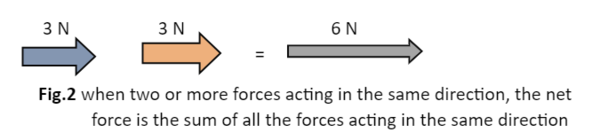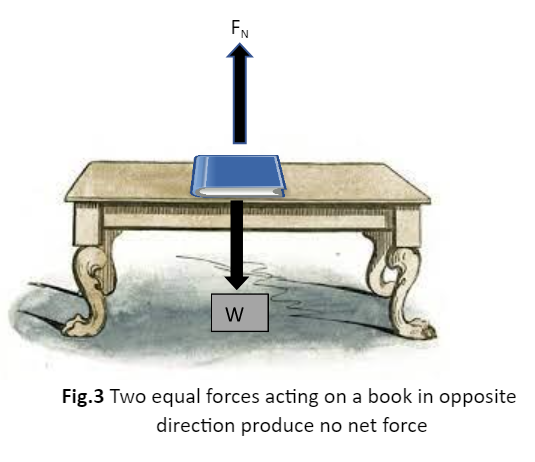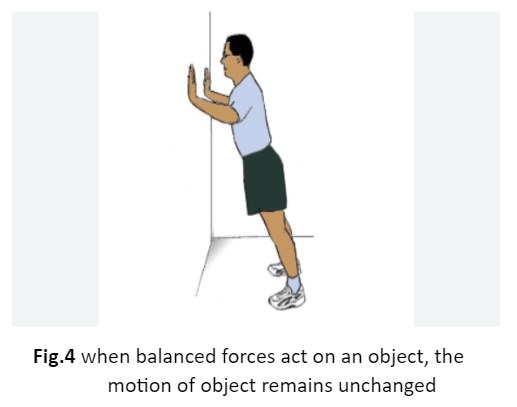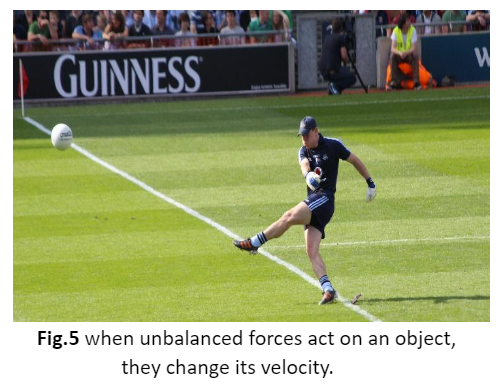• ### Browse All Lessons

##### Assign Lesson

Help Teaching subscribers can assign lessons to their students to review online!

 Tweet# Force

This lesson aligns with Next Generation Science Standards (NGSS) PS2.B

Introduction
A cyclist moves the pedal quicker when he climbs up the hill. He applies brakes as he reaches the top of the hill. Soon, he again starts to move in the opposite direction to climb down the hill. Something causes the cyclist to start moving, stop moving, or change direction. The external agent which causes the changes in the state of the cyclist is known as force. The force exerted on an object not only changes its state of motion or direction but can also change its shape. In this article, we will learn about force, the unit of force, net force, and balanced and unbalanced forces.

Force
The term force has a very simple and specific meaning that can be defined as “any push or pull on an object is considered a force”. When two objects interact with one another, one object pushes or pulls another object. we can say that the first object exerts a force on the second object to change its state of motion or state of rest. In other words, force is an influence that causes the change in the state of motion of an object.

For instance, we use force on wet clothes to squeeze all the water in them, we exert force to open or shut the door, and use force to squeeze a tube of toothpaste.Fig.1 we exert a force when opening a door

Formula of Force
Force is a vector quantity that is described by its magnitude and by the direction in which it acts. The quantity of force can be expressed by the vector product of mass and acceleration. Mathematically, it can be expressed as;

Force = mass$*$ acceleration
Force = $ma$

An arrow is used to represent the direction and strength of the force. The head of the arrow points in the direction of a force and the length of the arrow represents the strength of a force.

Unit of Force
The S.I. unit for the magnitude of the force is newton (N). English scientist and mathematician Isaac Newton was one of the first scientists who studied gravity and force. Therefore, the unit’s name is Newton. The force is also expressed in dyne.

Net Force
When more than one forces act on an object at once, the result produced is a combination of all the forces. The cumulative effect of all the forces acting on an object is known as the net force. The net force decides whether the object moves and also determines in which direction it moves.

Forces Acting in the Same Direction
When two or more forces act on an object in the same direction, the net force is equal to the sum of the individual forces. The net force acts in the same direction as the individual forces. In the figure below, the magnitudes of two forces are added together to find the net force.Forces Acting in the Opposite Direction
When two or more forces act on an object in the opposite direction, the net force is equal to the difference between the two forces. In other words, one force is subtracted from the other to find a net force just like adding a positive number to a negative number. The resulting force always acts in the direction of the greater force. If the strength of opposing forces is equal, they cancel each other and the net force is zero. For instance, a book lying on a table.Balanced and Unbalanced Forces

Balanced Forces
If equal forces act on an object in opposite direction, the resulting force of all the forces sums up to zero, the forces are known as balanced forces. In the case of balanced forces, the motion of a body remains unchanged. For example, a person is trying to push a wall as shown in the figure, but neither the wall moves nor the person. This is because both are exerting equal force in opposite directions on each other.Similarly, in a tug-of-war between two people, if each person exerts equal force to pull the rope, neither the rope nor the people will move.

Unbalanced Forces
When unbalanced forces act on an object, it will change its state of motion. The object can slow down, speed up or change direction. When the resultant force of all the forces acting on an object is not equal to zero, the forces acting on an object are known as unbalanced forces.

For instance, a player kicks a soccer ball and moves from one place to another. The movement of the ball represents that unbalanced forces are acting upon it.Summary
• Force is a push or pull on an object that changes its state of motion, state of rest, or its direction.
• The combination of all the forces acting on a body is known as the net force.
• If the resulting force of all the individual forces is equal to zero, the forces are known as balanced forces.
• If the sum of all the forces is not equal to zero, the forces are known as unbalanced forces.

Related Worksheets: